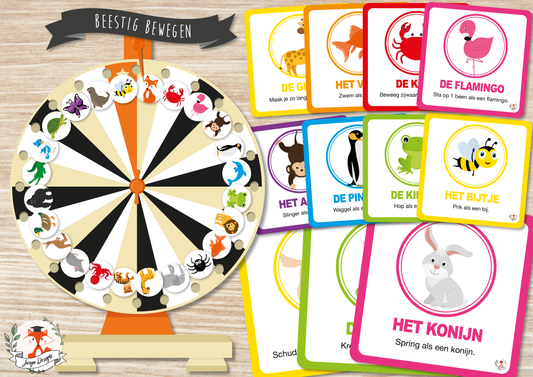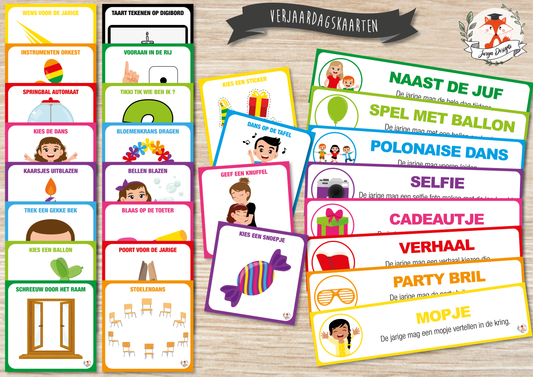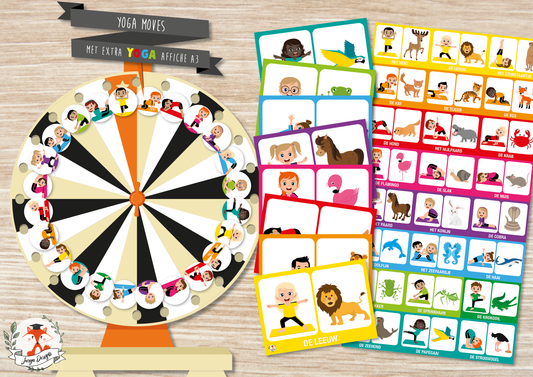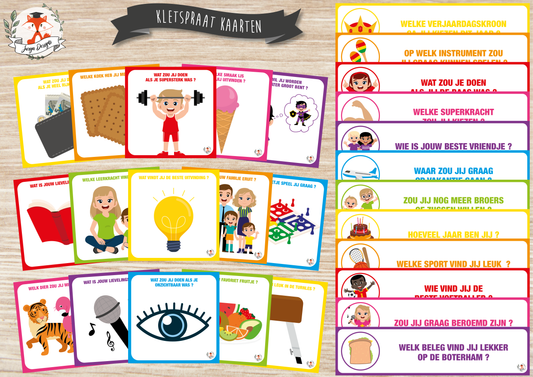BUNDEL5 = 10% | BUNDEL10 = 15% | BUNDEL20 = 20% | BUNDEL30 = 30% | BUNDEL40 = 40% | BUNDEL5 = 10% | BUNDEL10 = 15% | BUNDEL20 = 20% | BUNDEL30 = 30% | BUNDEL40 = 40% | BUNDEL5 = 10% | BUNDEL10 = 15% | BUNDEL20 = 20% | BUNDEL30 = 30% | BUNDEL40 = 40% | BUNDEL5 = 10% | BUNDEL10 = 15% | BUNDEL20 = 20% | BUNDEL30 = 30% | BUNDEL40 = 40% | BUNDEL5 = 10% | BUNDEL10 = 15% | BUNDEL20 = 20% | BUNDEL30 = 30% | BUNDEL40 = 40% | BUNDEL5 = 10% | BUNDEL10 = 15% | BUNDEL20 = 20% | BUNDEL30 = 30% | BUNDEL40 = 40% | BUNDEL5 = 10% | BUNDEL10 = 15% | BUNDEL20 = 20% | BUNDEL30 = 30% | BUNDEL40 = 40% | BUNDEL5 = 10% | BUNDEL10 = 15% | BUNDEL20 = 20% | BUNDEL30 = 30% | BUNDEL40 = 40%

• ### Animal Movements

Regular price \$6.00 USD
Regular price \$0.00 USD Sale price \$6.00 USD• ### Birthday cards

Regular price \$6.00 USD
Regular price \$0.00 USD Sale price \$6.00 USD• ### Yoga Moves

Regular price \$8.00 USD
Regular price Sale price \$8.00 USD• ### Chatter Talk Cards

Regular price \$8.00 USD
Regular price \$0.00 USD Sale price \$8.00 USD